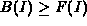Next: Design and Analysis of Up: Traffic Modeling Previous: The Network

## Maximum and Minimum Traffic Functions

We define the output traffic function Ri, X(t) of connection Mi at the (variable) server X to be the amount of data of Connection Mi departing from Server X during the time interval [0,t). Obviously, Ri, X(t) precisely describes the traffic of Connection Mi at the output of Server X. The fact that this function is time-dependent makes it an unlikely candidate for a traffic descriptor. We consider two more concise functions, which deterministically bound the expected traffic of a connection, and therefore can be used as envelope to characterize the traffic.

We call Function Fi, X(I) the maximum traffic function for Connection Mi at Server X if for any I > 0,

That is, the maximum amount of traffic output from Server X for Connection Mi during any time interval of length I is at most Fi, X(I).

Similarly, we define fi, X(I) to be the minimum traffic function. That is, for any I > 0,

Again, during any interval of length I, the amount of traffic output from server X for Connection Mi is at least fi,X(I).Figure 1 shows a related measure, the maximum rate functionof a traffic flow. In this example, a traffic stream is measured by a network analyzer as it enters an ATM network, and the maximum average rateis plotted as a function of the averaging interval I.

We use the functions F() and f() as traffic descriptors for the traffic of connections in the networks. F() and f() form the tightest deterministic time-invariant characterization of the traffic at the output of a server. As F() and f() may be defined by a large number of points, they are cumbersome to manipulate and bounds on the maximum and minimum traffic functions are used to characterize the traffic.

We define the maximum traffic bounding function B(I) to be an upper bound on F(I), that is,for all I. Similarly, we define the minimum traffic bounding function b(I) to be a lower bound on f(I), that is,for all I. Since we base our detection mechanism on B() and b(), the more tightly they bound the actual traffic, the more accurate is the resulting classification into compliant and non-compliant traffic.

In the context of real-time communication protocols, maximum traffic bounding functions are used to allocate resources, and tight bounding functions are sought to prevent excessive over-allocation of network resources. In practice, a trade-off must be made between tightness of the bounding function on one side, and the overhead incurred to manipulate it on the other, together with the inherent a-priori uncertainty about the traffic characteristics at the sources.

Traffic functions can be easily approximated with piecewise linear bounding functions at any level of resolution. Consider a maximum traffic function F(). Assume that we know one point of the function F(), that is, we know B' = F(I') for some value of I'. We then have a first-order approximation of F(I), which is given by

This can be recursively used to bound the function if more points are known. In this form, coarse bounds (three to five linear segments) on maximum traffic functions can be used for resource allocation purposes, where a broad categorization of traffic streams into classes - for example teleconference, or advertising, or sports - is sufficient. More accurate bounds (say, ten linear segments) can then be used to closely characterize individual traffic streams.

Once the traffic bounding functions are known at the entrance to the network, they can be derived for any point along the path of a connection. This derivation requires to obtain the traffic at the output of a server from the traffic at its input.

Let X and Y be two adjunct servers, and let Connection Mi first traverse Server X and then Server Y. Then,

Fi,Y(t) = Fi, X(t + d)

and

fi,Y(t) = fi, X(t - d)

where d is the worst-case delay experienced by Connection Mi at Server Y. The value for d depends on the scheduling methodology used in the server and on the traffic functions of other connections using that server. For a FCFS service discipline, for example, the worst-case delay on Server X can be bounded as follows, assuming that Server X serves N connections, and the traffic of a connection Mj is bounded at the output of the previous server by the maximum traffic bounding function Bj,PREV():

Various analytical techniques to derive worst-case delays based on traffic bounding functions for other scheduling policies, such as Static Priority, Generalized Processor Sharing, and various forms of Earliest-Due-Date have been proposed ([4, 5, 7, 8, 12] among many others). The above formula imply that, for Server Y, the upper and lower traffic at its output, modeled by Bi,Y(t) and bi,Y(t), can be derived from the traffic at its input (i.e., the output of the previous Server X).Figure 2 illustrates how the maximum and minimum traffic functions define an envelope for the amount of traffic on the connection at the output of a server. The network will, during run time, dynamically examine the traffic and verify if the traffic lies within this envelope. In the case of a violation is found, then a (potential) intrusion is detected. Actions can be taken to immediately suppresses the violation. These functionalities are implemented in a security device, which we discuss next.Next: Design and Analysis of Up: Traffic Modeling Previous: The Network
Riccardo Bettati
1999-02-23